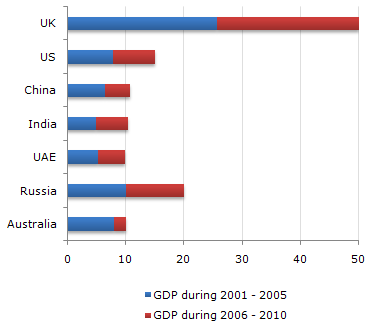# Data Interpretation - Bar Charts - Discussion

The following bar chart represents the GDP of different countries during the half decades 2001 - 2005 and 2006 - 2010. All figures are in Rs. billion.

GDP of Various Countries4.

Out of every Rs. 10,000 spent during the decade 2001 - 2010 approximately how much was the GDP of Russia during the half decade 2001 - 2005 ?

 [A]. Rs. 700 [B]. Rs. 1,400 [C]. Rs. 2,800 [D]. None of these

Explanation:

Russia will account for 10 out of 125, i.e, 8% of the total, i.e, 800 out of 10,000 (approximately values based on visual interpretation).

 Vandna said: (Aug 22, 2010) From where did it 125 come? Why in the answer its written 10 out of 125?

 Lkpoir said: (Aug 5, 2011) How to rate a chart? I mean what can be rated about the gdp of russia for example?

 Sriram said: (Sep 12, 2011) Can any body explain this puzzle?

 Bharath said: (Oct 5, 2011) The only way I could think of arriving at 125 is to approximately add all the gdp's from UK to Aus (50+15+10+10+10+20+10 = 125).

 Sachin said: (Sep 18, 2014) From where did it 125 come? Why in the answer its written 10 out of 125? Explain in detail.

 Manish said: (Oct 1, 2014) Guys Here is the answer: See the question here is a bit tricky: Its saying that if 10,000 is for whole for a decade for everyone. Than Russia's share for 5 years. So Include everything like Bharat said - (50+15+10+10+10+20+10 = 125). And than see the Bar: Russia's First 5 Years during the half decade 2001 - 2005 = 10 points so, We have 10 out of 125.

 Swapnil said: (Dec 7, 2015) Language of the question is a bit odd, especially "out of every 10000 spent" it should be different.

 Amy said: (Oct 28, 2020) Thanks for explaining @Manish.

 Sayli said: (Jan 12, 2021) Thanks for explaining.